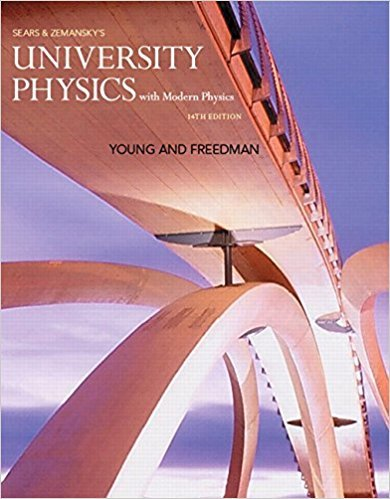×
Log in to StudySoup
Get Full Access to University Physics With Modern Physics (1) - 14 Edition - Chapter 29 - Problem Q29.4
Join StudySoup for FREE
Get Full Access to University Physics With Modern Physics (1) - 14 Edition - Chapter 29 - Problem Q29.4

Already have an account? Login here
×
Reset your password

# Solved: For Eq. (29.6), show that if v is in meters perISBN: 9780321973610 228

## Solution for problem Q29.4 Chapter 29

University Physics with Modern Physics (1) | 14th Edition

• Textbook Solutions
• 2901 Step-by-step solutions solved by professors and subject experts
• Get 24/7 help from StudySoup virtual teaching assistantsUniversity Physics with Modern Physics (1) | 14th Edition

4 5 1 292 Reviews
19
4
Problem Q29.4

For Eq. (29.6), show that if v is in meters per second, B in teslas, and L in meters, then the units of the right-hand side of the equation are joules per coulomb or volts (the correct SI units for E)

Step-by-Step Solution:
Step 1 of 3

Lab 2: Kinematics Members: Samantha Rubin, Marguerite Furlong, Zoe Gaber Table Number: 2 Introduction Kinematics​ explores the relationship between objects and motion, particularly for this lab, an object in free fall. Velocity and acceleration are mathematically represented with their derivatives in respect to either position or velocity in terms of time: Here, Δx is identified as the initial position of x subtracted from the final position of x, and Δv, is the initial velocity subtracted from the final velocity. When acceleration is constant with respect to time, we can relate the following kinema

Step 2 of 3

Step 3 of 3

##### ISBN: 9780321973610

Unlock Textbook Solution

Enter your email below to unlock your verified solution to:

Solved: For Eq. (29.6), show that if v is in meters per